# Problem 53975. Compute the effective conductivity of more heterogeneous aquifers

Cody Problem 52070 asked for a function to compute the effective hydraulic conductivity of a heterogeneous aquifer—or the single value of conductivity set such that the aquifer produces the same flow under the same total change in head. In that problem, the aquifer had soil units either in series only or in parallel only.
Write a function to compute the effective conductivity for two-dimensional flow in an aquifer with a more complicated distribution of conductivity. Flow is left to right, or to the east, as in the figure below. No flow occurs across the north and south boundaries. Assume the head difference is small enough that Darcy’s law applies. Use the conductivity specified on the equally-spaced grid provided.
For example, if in the aquifer below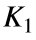= 0.1 m/d,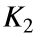= 0.2 m/d,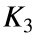= 0.01 m/d, and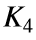= 20 m/d, then the effective conductivity is 0.092 m/d.
Hint: The simple formulas that work for soil units either in series only or in parallel only will not work for these more complicated distributions because two-dimensional flow violates assumptions behind the formulas. In this problem, compute the effective conductivity directly from the definition. Darcy's law yields the specific discharges (or flow per unit cross-sectional area) ofandThen conservation of mass leads toSolve this equation for the head h, compute the flow through the aquifer, and get the effective conductivity from the definition.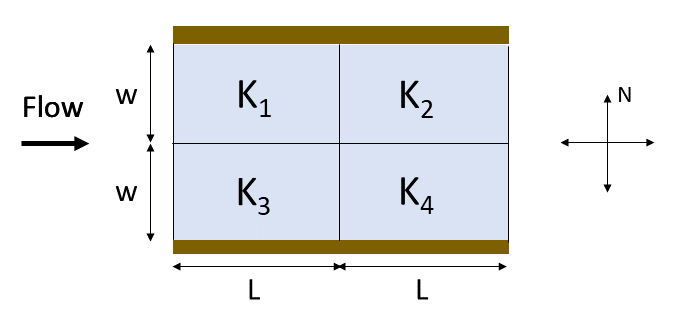### Solution Stats

50.0% Correct | 50.0% Incorrect
Last Solution submitted on Jan 31, 2022

### Community Treasure Hunt

Find the treasures in MATLAB Central and discover how the community can help you!

Start Hunting!